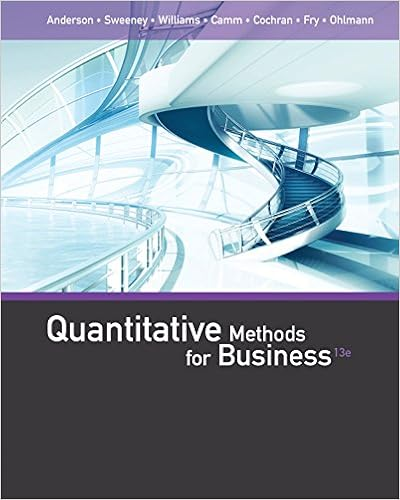# Be 11 hours and 009 hrs 2 can it be concluded at the

• Test Prep
• 5

This preview shows page 3 - 5 out of 5 pages.

##### We have textbook solutions for you!
The document you are viewing contains questions related to this textbook.The document you are viewing contains questions related to this textbook.
Chapter 3 / Exercise 15
Anderson/SweeneyExpert Verified
be 1.1 hours and 0.09 hrs2. Can it be concluded at the 5% level of significance thatthe supplier’s claim is incorrect?11. Three data entry specialists enter requisitions into a computer. Specialist A processes30% of the requisitions, Specialist B processes 45% and Specialist C processes 25%.IF 3% of the requisitions entered by A are incorrect, 5% of those entered by B areincorrect, and 2% of those entered by C are incorrecta) find the probability that a random requisition was entered incorrectly.b) If a random entry is found to be incorrect what is the probability that it was enteredby Specialist B?12. An aerospace company has submitted bids on two separate government contracts, Aand B. The company feels it has a 60% chance of winning contract A and a 30% chanceof winning contract B. If it wins contract B, the company believes it will have an 80%chance of winning contract A. What is the probability that the companya) will win both contracts?b) will win at least one of the two contracts?c) will not win either of the two contracts?d) Are the events “wins contract A” and “wins contract B” mutually exclusive? Whyor why not? Are they independent? Why or why not?13. Suppose it is known that the population standard deviation is 2.25. It is desired toestimate the population mean with a bound on the margin of error not exceeding 0.5unit with probability 0.95. How large a sample should be taken?14. IfXhas an exponential distribution with parameterλ, derive a general expression forthe (100p)th percentile of the distribution. Then specialize to obtain the median.15. Find the joint and marginal densities corresponding to the cdfF(x, y) = (1-e-x)(1-e-βy)x0y0>0β>0.16. Let the joint probability function ofXandYbef(x, y) =(e-(x+y)0< x <1,0< y <10otherwise.a)FindP(X >1)b)FindE(Y)c)AreXandYindependent random variables?3
##### We have textbook solutions for you!
The document you are viewing contains questions related to this textbook.The document you are viewing contains questions related to this textbook.
Chapter 3 / Exercise 15Expert Verified
•••